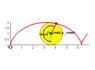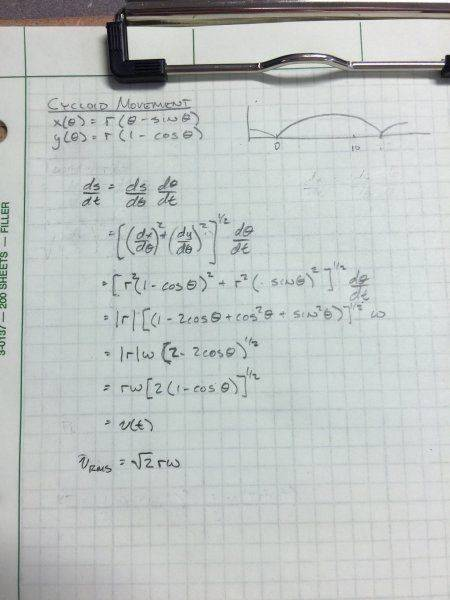# Inertia effects of components on a car vs mass fixed in car

• Automotive
• zanick

#### zanick

This is a pretty hot and rarely understood topic in the automotive / racing industry.
Sure, everyone knows spinning mass on a car is worse than mass sitting in a car. its effects are greater, but by how much? Besides the benefits of the unsprung weight having less gyroscopic forces effecting handling, its effect on acceleration is much less than most understand or realize. often times, racers will use a 5 lb lighter wheel and somehow conclude that they saved 2 seconds a lap due to this type of weight savings.
I always like to challenge even the gyroscopic effects because In most cases, on a road course, the steering wheel is only rotating 90 to 180 degrees max. the angle of the tire, might only move a few degrees. :)

Anyway, the weight on the tire or circumference has an effect of 2x that of if that same weight was sitting in the car, looking strictly on the force needed to accelerate it.
the rotational equations are the familiar , (one for inertia) I =MR^2 and then for the (one for Kinetic energy) KE=1/2Iw^2

If you do a translational vs rotational comparison, If the weight is at the circumference, tied to the center with a massless connection the effects of the rotating mass plus the linear movement (non slipping) would be exactly 2 x that if the weight was in the car. if we are talking wheels , or things that don't have the same weight distribution as a hollow cylinder, then the formula works out to provide an answer that is much less.
1.4 x if that weight was on the rim for example, as if it was in the car.
This is very hard to swallow for most racers, who generally have to blame something other than their driving for slower times, or are so proud of their modifications, that they over credit them. Racers are a stranger breed... I know, I'm one of them. so when a racer finds out that his 2lbs of tire weight x 4 is only equal to 8lbs rotating, or 16 lbs as if it was sitting in the car. hardly enough to worry or give any credit for, and as I mentioned, its effect on handling is going to something, but hardly enough to feel in reality. certainly won't be responsible for any measurable faster accelerations or as some thing in the street word, better fuel economy, as we all know, If there is no change in speed (like a MPG test) there is no effect of that weight vs it sitting in the car and 8lbs in the car (like a small bag of groceries) is not going to effect rolling friction to change MPG, though many swear that it does... :)

anyway, my question is about the actual physics equations and how they are validated or explained. Not just the math... I get that, I am talking about real reasons.
for example, basically, when you are talking about rotating mass in a hollow cylinder, its the same as if a solid mass was on a massless connection (radius) to the center. that hollow cylinder would be rolling and the KE linear plus the KE rotationaly is added together to get the total KE and this is double of the KE if it was just flying in a straight line through space.
How do we account for that. Plotting the actual movement of a mass on the end of a string with a given radius, it only looks like it travels about 25% further than if the mass was at the same linear velocity but not spinning. So it has to be traveling approx 25% faster, so going through the rough calculations, 1/2mv2 of something going 25% further , is only 1.8 times the KE, and not the 2x the equations produce. Why is that? it first seems that there is a little redundancy in the equations being added together when you look at the picture below and see what the mass is actually doing through rotation and linear movement combined. Are there other forces that I might not be considering?

#### Attachments

•wheel.png
8.2 KB · Views: 492
Last edited:

Here's my attempt at an explanation:

The equivalent point mass of the wheel is traveling along a cycloid path. The speed at which it's traveling I calculated is given by the function ##v(t)=r\omega \sqrt{2(1-\cos{\theta})}## for ##\theta \in [0,2\pi]##. I figured a good average value would be the RMS speed. If you take the root mean square, you get ##v_{RMS}=\sqrt{2}r\omega##. Plugging that into the translational kinetic energy equation, ##KE=\frac{mv_{RMS}^2}{2}=\frac{m(2r^2\omega^2)}{2}=mr^2\omega^2##. If we assume no slip in the wheels, then we can say that ##v=r\omega## and also ##mv^2=mr^2\omega^2##.

If we look at the wheel with the rotational/translational method, ##KE=\frac{mv^2}{2}+\frac{I\omega^2}{2}##. Since this is a thin tube, ##KE=\frac{mv^2+mr^2\omega^2}{2}=\frac{2mv^2}{2}=mv^2##.

My derivation for the point mass speed along the cycloid path is as follows:As far as blaming external factors for their race times, I think that the racers tend to be a bit superstitious. I know a couple street racers and they swear up and down that the spoiler on their import tuners gives them an extra couple HP. :)

Last edited:
Assuming the wheel is always rolling without slipping, you can figure out what i call effective mass.
You need the mass moment of inertia of the wheel, the mass, and the radius.
Do an example constant speed (i use 10 m/s) for the wheel, figure out the KE rotating and KE linear at this speed.
Then apply to this equation:
effective mass = ( 1 + ( KE rotating / KE linear ) ) * actual mass

Are there other forces that I might not be considering?

No. What you are not considering is the true mathematical definition:

$$E \ne \frac{1}{2}mv^2$$

$$E = \int mvdv$$

ONLY if $m$ is constant and $v$ varies from 0 to $v_x$ linearly, that you can do this:

$$E= m \int_0^{v_x} vdv = m \left[ \frac{1}{2}(v_x)^2 - \frac{1}{2}(0)^2 \right] = \frac{1}{2}mv_x^2$$

Jack...it is just not worth it...no way am i going to get in this tangled deluded, misinformed, incorrect and illogical thread that is biased against my fellow racers...every premise is misinformed and ill conceived ..it makes my hair hurt

The unsprung weight of the wheels and tires (versus the weight of the rest of a car) has an effect on how well the tires can follow irregularties in the surface of a race track. Part of this is due to the fact that the lighter the wheels and tires, the less the rest of the car is disturbed by track irregularties. This is probably the main reason for lighter wheels and tires on race cars.

In the case of bicycles used for velodrome racing, there's a burst of acceleration near the end of a race to the top speed that the riders can acheive, and the competitors fill the tires with helium instead of air, just for that slight advantage in acceleration.

The linear KE and rotational KE would only be the same if the wheel was a thin hoop with all the mass concentrated in it (never happens), all real wheels have the mass distributed more evenly.